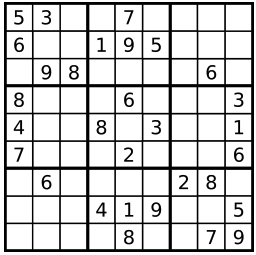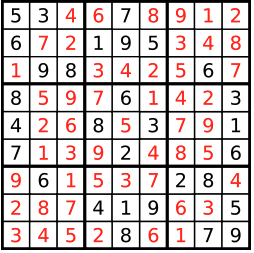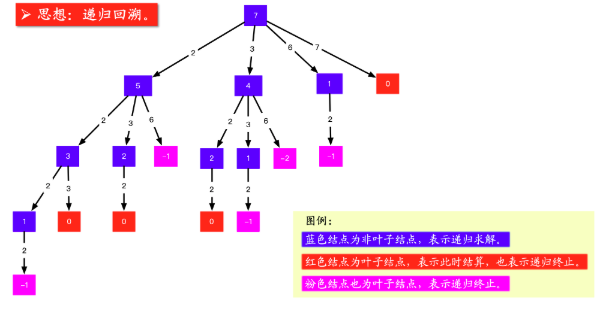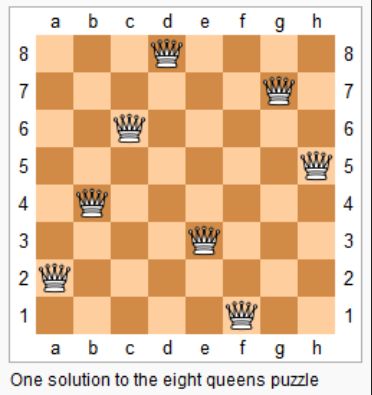# 2. 解题

## 2.1. 解数独

### 2.1.1. 描述

1. 数字 1-9 在每一行只能出现一次。
2. 数字 1-9 在每一列只能出现一次。
3. 数字 1-9 在每一个以粗实线分隔的 3x3 宫内只能出现一次。## 2.2. 组合总和

### 2.2.1. 描述

candidates 中的数字可以无限制重复被选取。

• 所有数字（包括 target）都是正整数。
• 解集不能包含重复的组合。

``````输入: candidates = [2,3,6,7], target = 7,

[
,
[2,2,3]
]
``````

``````输入: candidates = [2,3,5], target = 8,

[
[2,2,2,2],
[2,3,3],
[3,5]
]
``````

### 2.2.2. 解题思路## 2.3. 通配符匹配

### 2.3.1. 描述

``````'?' 可以匹配任何单个字符。
'*' 可以匹配任意字符串（包括空字符串）。
``````

• s 可能为空，且只包含从 a-z 的小写字母。
• p 可能为空，且只包含从 a-z 的小写字母，以及字符 ? 和 *。

``````输入:
s = "aa"
p = "a"

``````

``````输入:
s = "aa"
p = "*"

``````

``````输入:
s = "cb"
p = "?a"

``````

``````输入:
p = "*a*b"

``````

``````输入:
s = "acdcb"
p = "a*c?b"

``````

## 2.4. N皇后

### 2.4.1. 描述

n 皇后问题研究的是如何将 n 个皇后放置在 n×n 的棋盘上，并且使皇后彼此之间不能相互攻击。``````输入: 4

[".Q..",  // 解法 1
"...Q",
"Q...",
"..Q."],

["..Q.",  // 解法 2
"Q...",
"...Q",
".Q.."]
]

``````